GFG App
Open AppBrowser
Continue

# How to Find the Intersection Between a Curve and Straight Line in Excel?

When two lines or curves having two different equations intersect with each other at one common point is known as Intersection. We can represent the point of intersection in Excel and also we can find the point of intersection using Excel functions SLOPE and INTERCEPT

Behind the intersection of a curve and a straight line two mathematical reasons are present:

1. The value of y1 is always lesser than the value of y2 at a given x before the intersection of lines.
2. The value of y1 is always lesser than the value of y2 at the next value of x (xi+1) before the intersection of lines.

Follow the further steps to find the intersection between a curve of equation y2=x^2+2 and a straight line of equation y1=2x+4.

### Step-by-Step Implementation

Step 1: Find the values of y1 and y2 for different x values.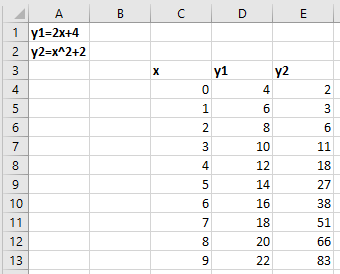Step 2: Calculate the difference between y1 and y2.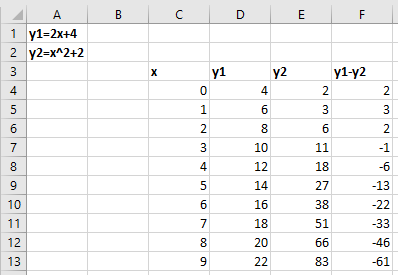Step 3: We have to calculate the point of intersection using

=IF(SIGN(F4*F5)=1,0,1),

where the value will be 1 the point of intersection will be that.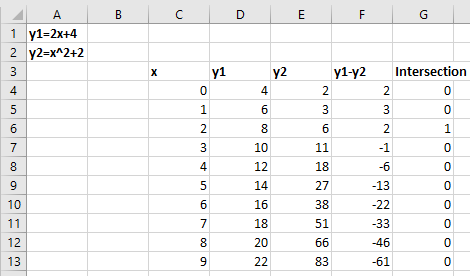G13 has to consider zero because the next cell is empty.

Step 4: The value of x between which two lines have crossed can be calculated by

=MATCH(1,G4:G13,0)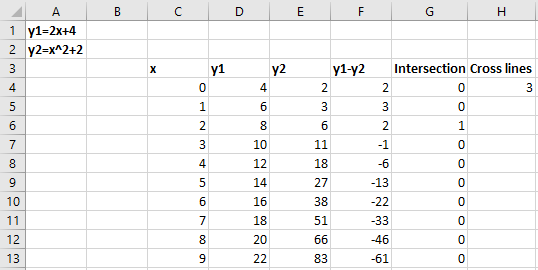Step 5: X’s and Y’s data points have to be calculated by

=INDEX(C4:C13, H4)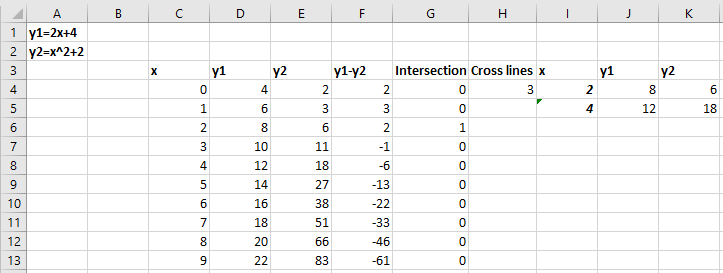Step 6: Slope and Intercept can be calculated by

=SLOPE(J4:J5, I4:I5)

=INTERCEPT(J4:J5, I4:I5)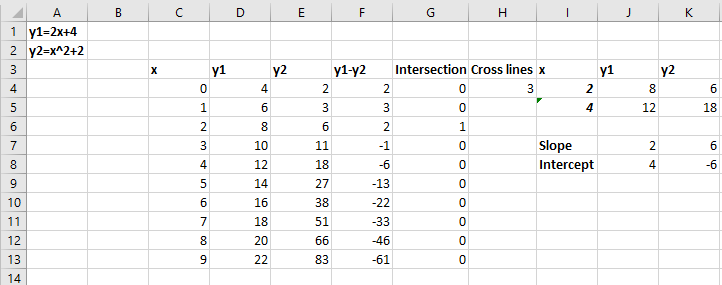Step 7: Plot the graph using scatter plot by selecting the Insert tab and then selecting scatter in the chart section.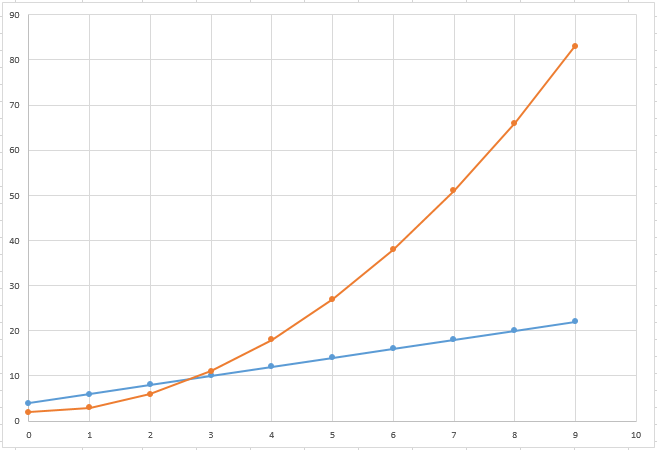Here, the Blue line is for a straight line(y1) and the orange line is for a curve(y2).

My Personal Notes arrow_drop_up